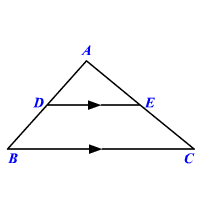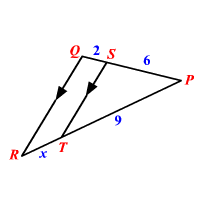# Triangle Proportionality Theorem

If a line parallel to one side of a triangle intersects the other two sides of the triangle, then the line divides these two sides proportionally.If $\stackrel{¯}{DE}\parallel \stackrel{¯}{BC}$ , then $\frac{AD}{DB}=\frac{AE}{EC}$ .

Example :

Find the value of $x$ .The lines $\stackrel{¯}{QR}\text{\hspace{0.17em}}\text{\hspace{0.17em}}\text{and}\text{\hspace{0.17em}}\text{\hspace{0.17em}}\stackrel{¯}{ST}$ are parallel.

Therefore, by the Triangle Proportionality Theorem,

$\frac{PS}{QS}=\frac{PT}{RT}$

Substitute the values and solve for $x$ .

$\frac{6}{2}=\frac{9}{x}$

Cross multiply.

$6x=18$

Divide both sides by $6$ .

$\begin{array}{l}\frac{6x}{6}=\frac{18}{6}\\ x=3\end{array}$

The value of $x$ is $3$ .